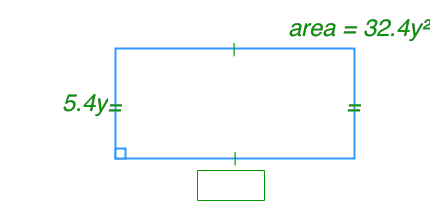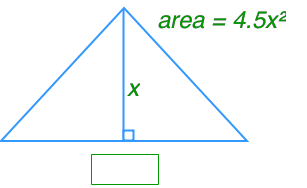# Multiplying and dividing monomials

### Multiplying and dividing monomials

In this section, we will practice multiplying and dividing polynomials. Furthermore, we will use polynomial multiplication and division to solve unknowns such as, side length, and surface area, of polygons.

#### Lessons

• Introduction
a)
How to multiply monomials?

b)
How to divide monomials?

• 1.
Model and calculate each multiplication.
a)
$\left( {6x} \right)\left( {2x} \right)$

b)
$\left( { - 4y} \right)\left( {3y} \right)$

c)
$\left( x \right)\left( { - 5x} \right)$

d)
$\left( { - 2x} \right)\left( {7x} \right)$

• 2.
Multiply.
a)
$\left( x \right)\left( { - 4x} \right)$

b)
$\left( {5x} \right)\left( {3x} \right)$

c)
$\left( { - 2x} \right)\left( { - 6x} \right)$

d)
$\left( { - 3.1x} \right)\left( { - 4x} \right)$

e)
$\left( { - \frac{3}{4}x} \right)\left( {16x} \right)$

f)
$\left( { - 8x} \right)\left( {2.3y} \right)$

• 3.
Divide.
a)
$\frac{{144x}}{6}$

b)
$\frac{{84xy}}{{4xy}}$

c)
$\frac{{9{x^2}}}{x}$

d)
$\frac{{18xy}}{{3x}}$

e)
$\frac{9.6{p^2}}{ - 8p}$

f)
$\frac{{ - 25x}}{{ - 5x}}$

• 4.
Find the missing dimension.
a)b)